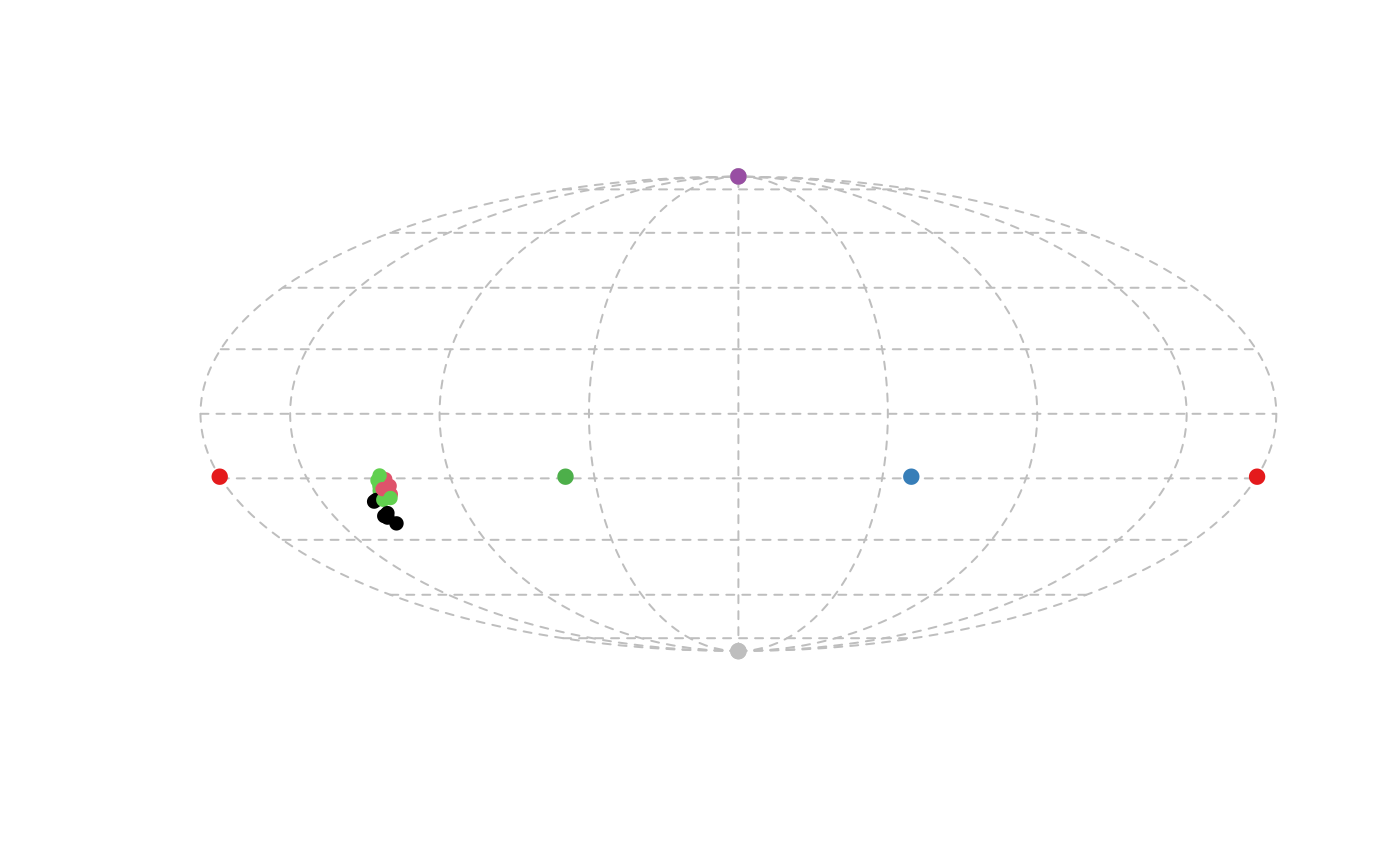Produces a 2D projection plot of points in a tetrahedral colour space

Adds points to a tetrahedral colorspace projection

projplot(tcsdata, ...)

projpoints(tcsdata, ...)

## Arguments

tcsdata

(required) tetrahedral color space coordinates, possibly a result from colspace(), containing values for the 'h.theta' and 'h.phi' coordinates as columns (labeled as such).

...

additional parameters to be passed to the plotting of data points.

## Value

projplot() creates a 2D plot of color points projected from the tetrahedron to its encapsulating sphere, and is ideal to visualize differences in hue.

projpoints() creates points in a projection color space plot produced by projplot().

## Note

projplot() uses the Mollweide projection, and not the Robinson projection, which has been used in the past. Among other advantages, the Mollweide projection preserves area relationships within latitudes without distortion.

## Author

Rafael Maia rm72@zips.uakron.edu

## Examples

data(sicalis)
vis.sicalis <- vismodel(sicalis, visual = "avg.uv")
tcs.sicalis <- colspace(vis.sicalis, space = "tcs")
projplot(tcs.sicalis, pch = 16, col = setNames(rep(seq_len(3), 7), rep(c("C", "T", "B"), 7)))